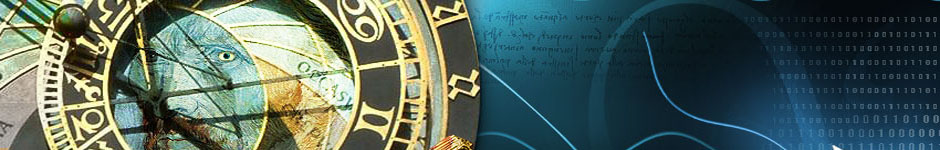## Irrational Numbers Are Stupid and Do Not ExistIn this post, I will be making the claim that all numbers are rational. Please excuse the math, and try to follow along if you can. Without further ado, let us examine this idea further.

1 is rational.
.5 is rational.
.3333… is rational.

What does it mean for these numbers to be rational?  A number x is rational if it fits the definition:

x = a/b where a and b are whole numbers and b is not equal to zero.

Using this as our definition, we may substitute whole numbers for a and b to create an array of rational numbers.  For example

Let a = 1 and b = 1.  Then x = 1/1 = 1 = a rational number.
Let a = 1 and b = 2.  Then x = 1/2 = .5 = a rational number.
Let a = 1 and b = 3.  Then x = 1/3 = .3333… = a rational number.

What are the distinct characteristics of rational numbers?  They typically terminate and repeat indefinately with a discernable pattern ( we usually leave out the extra zeros out to save money on ink ).  Incidentally, any number that fits the description I have just given may be converted back into a fraction with by following process:

x = .4545….
100 * x = 45.4545…
100x – x = 45.4545… – .4545…
99x = 45
Then x = 45/99 = .4545… = a rational number.

(If the length of the repetition of digits after the decimal place is longer or shorter, you can alter the power of ten used in the multiplication operation and match the number of zeros in that power of ten to the length of repetition).

What does it mean for a number to be irrational?  A number x is said to be irrational if we are unable to express it in the fractional form defined above.  Some examples of numbers traditionally  considered to be irrational:

pi = 3.1415926535…..
sq(2) = 1.4142135623…
e = 2.7182818284…

Why are we unable to prove these numbers are rational?  Well, they repeat indefinately, but we are unable to discern any pattern from their digits.  For The purposes of this proof, allow me to reference the all-knowing wikipedia so that we may examine pi more closely:

“The first one million digits of π and 1/π are available from Project Gutenberg (see external links below). The current record (December 2002) by Yasumasa Kanada of Tokyo University stands at 1,241,100,000,000 digits, which were computed in September 2002 on a 64-node Hitachi supercomputer with 1 terabyte of main memory, which carries out 2 trillion operations per second, nearly twice as many as the computer used for the previous record (206 billion digits).”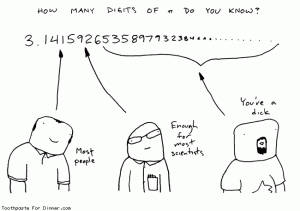Many would claim this as sufficient proof of irrationality and call off the search.  They have been unable to find any repeating pattern so they do not believe a pattern exists.  Yet these same individuals have chosen to discard all previous evidence and knowledge of the world in which we live.  They have chosen to accept a world in which irrational numbers exist.  A universe filled with irrational numbers is a cold, dark, scary place completely void of reason.  It is not a place in which we would want to raise our children.  We must all remember that fractions are our friends.  It is time for all the pessimists and lame-footed quitters to wise up to the real problem at hand.

The current approximation is only on the order of 10^13 digits.  This is a significantly insignificant amount.  We have not even scratched the surface of pi in our search for a pattern.  I have three points to make.  Three points I have, and the number of points to be made is threee.  Fully understanding these three points will allow you to understand why the universe must be rational.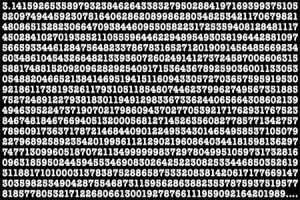Case One: We have not searched long enough.

Pi is finite and rational.  Given enough time, the proper formulas, and sufficient computing power, a repeating value for pi will be determined or it will terminate with a string of zeros.  We could then represent pi as a simple fraction of two whole numbers and start using our supercomputers for something a little more useful like videogames.  Frankly, I find this case to be highly unlikely.  If we DO find a fractional value for pi using the current methods, it will most likely mean that our formulas are wrong.  An accurate formulation is one which describes thing as they really are.  The formulas used to calculate pi have been proven wrong in the past and it will happen again.  To put things in perspective, people used to think pi was 22/7.  This has long since been shown to be false.  Accurate formulas are a necessary prerequisite for the accurate determination of pi. This brings me to my second point.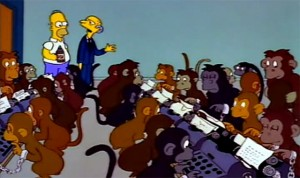Case Two: Some numbers are really big and very weird.

Pi is infinite and therefore irrational.  The emergence of inexplicable patterns is an inevitability when dealing with extremely large numbers.  This is a purely (il)logical result of dealing with the infinite.  Infinite numbers go on forever.  Forever is a very long time.  Given infinite time, Infinite monkeys hitting random keys on infinite typewriters would reproduce the entire works of Shakespeare.  Heck, you don’t even need infinite time for that.  Maybe just a couple of months if the monkeys worked diligently.  Granted, it would take a long time to sift through the noise and find the right string of letters.  Hamlet and Caesar would be hiding in there somewhere as long as you knew what you were looking for and where to look for it.  For the very same reason I could say with near-certainty that a refrigerator full of tofu created from completely natural and random processes is currently orbiting a neutron star 78 trillion trillion trillion light years away from earth, due to the immensity of the surface area of the selection sphere.  Just some random convergence of matter/energy spewed forth from the bowels of reality in the face of all reason.  I could even take a picture of it for you if only my telescope were big enough.

Sometimes it seems that things that immnese are just plain weird and we will have to leave it at that.  Maybe pi is infinite.  Maybe it is irrational.  Maybe the universe is irrational.  But you’ve read this far so you’re obviously not a quitter.  And you must certainly be intelligent enough to understand this next part.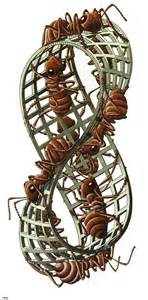Case Three.142:  Mathematics is unsound and our understanding of reality is quite limited.

Pi is both infinite and rational.  Using current deterministic methods, the best we can say about the rationality of pi is that it is…well…undetermined.  We may not assume that irrational numbers exist.  Our definition for what constitutes a rational numbers x is wrong, or, at the very least, arbitrary.  Consider the following for just a moment.

Change the definition of a rational number to allow b = 0.
Then x = a / 0 = infinity = a rational number.

How does this relate to pi?  Well, specifically, the notions of Euclidean Geometry seem to be leading us astray when combined with simple Algebra.  The interactions between points and lines on a 2-dimensional membrane of zero thickness are strange.  They may  serve adequately to describe one’s immediate surroundings over the course of a normal day, but they are ultimately founded on misguided assertions that do not coincide with the true nature of reality and crumble under closer scrutiny.  There, I said it!  Euclidean Geomety seems to lead us invariably to the conclusion that pi is irrational.  However!  Euclidean Geometry also exists completely within the realm of imagination.  Therefore, we have imagined something which is irrational and meaningless.  This Geometry we use is only an approximation of reality based on a limited perception of events.  I would assume my own beliefs and perceptions were flawed before judging the universe to be broken.  All we have to do in order to fix our problem is change the way we think.  Maybe space is curved.  Maybe 2-dimensional objects exist in a higher-dimensional space.  Maybe the universe as we see it exists only on the surface of a Moebius strip.  Maybe time runs both ways.  Maybe there is only one sun and source of light in the universe and the stars we see at night are but dim reflections painted on the ceiling of the world.  Maybe there are an infinite number of parallel realities which exist in a state of universal simultaneous entanglement.  We might conclude the existence of some odd quantum state of infinity so large that pi repeats and becomes rational as long as you dont observe it too closely, in which case a welcome to the strange world of quantum mathematics is in order.

« Previous: Principal Motivation

This site uses Akismet to reduce spam. Learn how your comment data is processed.